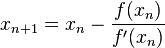# GATE | GATE-CS-2002 | Question 40

The Newton-Raphson iteration Xn + 1 = (Xn/2) + 3/(2Xn) can be used to solve the equation
(A) X2 = 3
(B) X3 = 3
(C) X2 = 2
(D) X3 = 2

Explanation: In Newton-Raphson’s method, We use the following formula to get the next value of f(x). f'(x) is derivative of f(x).Option (A)

```X2 = 3
f(x) = X2 - 3

Xn + 1 = Xn - (Xn2 - 3) / (2*Xn)
=  (Xn/2) + 3/(2xn) ```
My Personal Notes arrow_drop_up
Article Tags :

Be the First to upvote.

Please write to us at contribute@geeksforgeeks.org to report any issue with the above content.